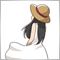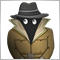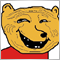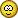# Is mql4 code stable?71

Hi, I am having trouble with my code and am wondering if the fault is always with the programmer or sometimes it could be the stability of mql4? The problems usually occur after a long list of "if statements". I've included a typical example of the complexity of the code below. There would be about 7 or 8 "if statements" in a row and should only return(true) if all are correct. But sometimes a statement is wrong but it still returns (true). I even have checked with the data window and one of  the statements would be definitely wrong. Anyway, what I am basically asking is do I assume there is always a mistake in my code or can the computer sometimes go haywire from reading the code. I should add that this is on a demo account but I don't see how that should change anything. Thanks

``` bool Scenario2_B()
{

if(High - Close < 30*Point)

if(Ema20_1 -20*Point > Ema100_1)
if(Ema20_1 > Ema20_40 || Ema20_1 > Ema20_60)
if(Ema100_1 > Ema100_20 || Ema100_1 > Ema100_40)
if(TimeCurrent() -1800 > TStamp2_B)
{
if((PivotAlign_1(Ema100_0,10*Point) == true)
|| (PivotAlign_2(Ema100_0,10*Point) == true)
|| (PivotAlign_3(Ema100_0,10*Point) == true))

if (Low -15*Point < Ema100_1)
if (MarketInfo(Symbol(), MODE_BID) > Ema100_0)
{
return(true);
}

else if((PivotAlign_1(Ema125_0,10*Point) == true)
|| (PivotAlign_2(Ema125_0,10*Point) == true)
|| (PivotAlign_3(Ema125_0,10*Point) == true))

if (Low -15*Point < Ema125_1)
if (MarketInfo(Symbol(), MODE_BID) > Ema125_0)
{
return(true);
}
}
return(false);
}```525

You need to clarify the scope of each if statement with { }.

For example.

```bool Scenario2_B()
{
if(High-Close<30*Point)
{
if(Ema20_1 -20*Point>Ema100_1)
{
if(Ema20_1>Ema20_40 || Ema20_1>Ema20_60)
{
if(Ema100_1>Ema100_20 || Ema100_1>Ema100_40)
{
if(TimeCurrent() -1800>TStamp2_B)
{
{
if((PivotAlign_1(Ema100_0,10*Point) == true)
|| (PivotAlign_2(Ema100_0,10*Point) == true)
|| (PivotAlign_3(Ema100_0,10*Point) == true))
{
if(Low-15*Point<Ema100_1)
{
if(MarketInfo(Symbol(),MODE_BID)>Ema100_0)
{
return(true);
}
}
}
else if((PivotAlign_1(Ema125_0,10*Point)==true)
|| (PivotAlign_2(Ema125_0,10*Point) == true)
|| (PivotAlign_3(Ema125_0,10*Point) == true))
{
if(Low-15*Point<Ema125_1)
{
if(MarketInfo(Symbol(),MODE_BID)>Ema125_0)
{
return(true);
}
}
}
}
}
}
}
}
}

return(false);
}
```38685

Mark Boc:

Hi, I am having trouble with my code and am wondering if the fault is always with the programmer or sometimes it could be the stability of mql4? The problems usually occur after a long list of "if statements". I've included a typical example of the complexity of the code below. There would be about 7 or 8 "if statements" in a row and should only return(true) if all are correct. But sometimes a statement is wrong but it still returns (true). I even have checked with the data window and one of  the statements would be definitely wrong. Anyway, what I am basically asking is do I assume there is always a mistake in my code or can the computer sometimes go haywire from reading the code. I should add that this is on a demo account but I don't see how that should change anything. Thanks

For this kind of problem : always in your code.7879

{} correctly put

``` bool Scenario2_B()
{
if(High - Close < 30*Point)
if(Ema20_1 -20*Point > Ema100_1)
if(Ema20_1 > Ema20_40 || Ema20_1 > Ema20_60)
if(Ema100_1 > Ema100_20 || Ema100_1 > Ema100_40)
if(TimeCurrent() -1800 > TStamp2_B)
{
if((PivotAlign_1(Ema100_0,10*Point))
|| (PivotAlign_2(Ema100_0,10*Point))
|| (PivotAlign_3(Ema100_0,10*Point)))
{
if (Low -15*Point < Ema100_1)
if (MarketInfo(Symbol(), MODE_BID) > Ema100_0)
return(true);

}
else
if((PivotAlign_1(Ema125_0,10*Point))
|| (PivotAlign_2(Ema125_0,10*Point))
|| (PivotAlign_3(Ema125_0,10*Point)))
{
if (Low -15*Point < Ema125_1)
if (MarketInfo(Symbol(), MODE_BID) > Ema125_0)
return(true);
}
}
return(false);
}```1079

I see nothing wrong in this code, the problem is probably in Ema* and PivotAlign* functions. Which statement was wrong?

In my cases it also has been the programmer. Every single time.7879

kypa:

I see nothing wrong in this code, the problem is probably in Ema* and PivotAlign* functions. Which statement was wrong?

In my cases it also has been the programmer. Every single time.

Find the 2 differences```      if(Buyticket2_4 == 0)
{
if((PivotAlign_1(Ema100_0,10*Point) == true)
|| (PivotAlign_2(Ema100_0,10*Point) == true)
|| (PivotAlign_3(Ema100_0,10*Point) == true))

if (Low -15*Point < Ema100_1)
if (MarketInfo(Symbol(), MODE_BID) > Ema100_0)
{
return(true);
}

else if((PivotAlign_1(Ema125_0,10*Point) == true)
|| (PivotAlign_2(Ema125_0,10*Point) == true)
|| (PivotAlign_3(Ema125_0,10*Point) == true))

if (Low -15*Point < Ema125_1)
if (MarketInfo(Symbol(), MODE_BID) > Ema125_0)
{
return(true);
}
}```
and
```                if(Buyticket2_4 == 0)
{
if((PivotAlign_1(Ema100_0,10*Point))
|| (PivotAlign_2(Ema100_0,10*Point))
|| (PivotAlign_3(Ema100_0,10*Point)))
{
if (Low -15*Point < Ema100_1)
if (MarketInfo(Symbol(), MODE_BID) > Ema100_0)
return(true);

}
else
if((PivotAlign_1(Ema125_0,10*Point))
|| (PivotAlign_2(Ema125_0,10*Point))
|| (PivotAlign_3(Ema125_0,10*Point)))
{
if (Low -15*Point < Ema125_1)
if (MarketInfo(Symbol(), MODE_BID) > Ema125_0)
return(true);
}
}```1079

The 'else' is about the second 'if' vs. the fourth and short writing for checking boolean functions?

I assumed he wants the 'else' on the last 'if', but it really makes more sense ema100 or ema125, not half of both.

P.S.71

Thanks guys for all your comments! Gives me a lot to go through but it is good to know the problem is with my code and not the system, so eventually I can figure it out.

Thanks!71

kypa:

The 'else' is about the second 'if' vs. the fourth and short writing for checking boolean functions?

I assumed he wants the 'else' on the last 'if', but it really makes more sense ema100 or ema125, not half of both.

P.S.